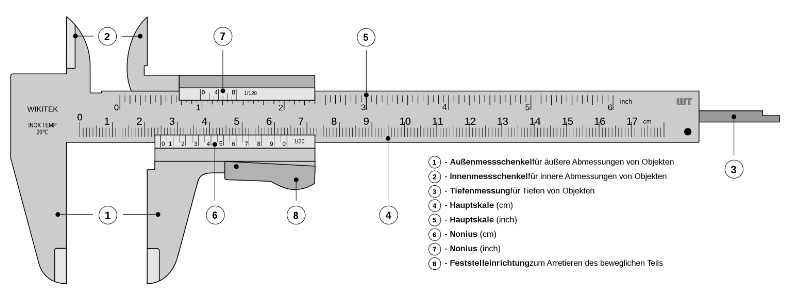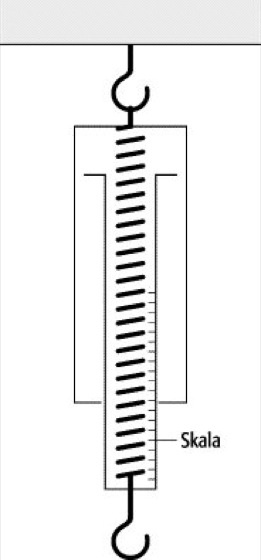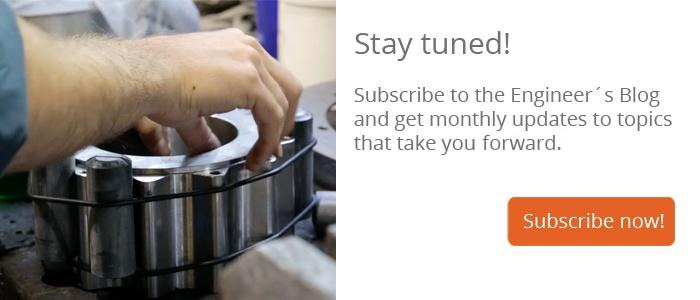Some weeks ago, we addressed the historical development of measurement technology. Today we would like to discuss the different measuring techniques and methods which help determine various parameters of a part being measured. Let's start with a few basic terms before we give a short overview of the most common measuring methods.By Vernier caliper legends.svg: Joaquim Alves Gaspar, vectorised by Antilivedderivative work: Sebastian Wallroth - Vernier caliper legends.svg, CC BY 2.5

## Metrology

The fundamentals of metrology are described in the standard DIN 1319-1:1995-01. Measuring techniques are based on the so-called “Metrology” that studies the scientific basics for the development of measuring instruments and their application. The metrological findings are implemented in the technology of measuring instruments and their use.

## Direct and indirect measuring methods

In the direct method of measurement, the quantity to be measured is compared directly with a standard (an agreed unit of measurement), i.e. the value is determined directly on the part being measured. Examples in this context are length comparisons with a scale, or mass comparisons with balance weights like a beam balance. Other typical examples of direct measurement procedures are the measurement of voltage or electric current, and the measurement of the temperature with a mercury thermometer.

Hence, the direct measurement procedure is a direct comparison and is, therefore, also called comparison method. The required international “basic units” are defined in the International System of Units (French: SI, Système international d’unités). This is a metric system of units and covers the following basic units from which all other units are derived:

• Length (1 m)
• Mass (1 kg)
• Time (1s)
• Electric current (1 A)
• Temperature (1K)
• Luminous intensity (1cd)
• Amount of substance (1mol).

The definitions of the basic units were subject to changes in the past. Up to 1960, for example, the primary meter was used as a prototype for the unit meter. Apart from the kilogram, all other units are meanwhile determined by unchangeable natural constants. Since the mass of the primary kilogram could theoretically change (and is likely to do so), research is being conducted to also unambiguously define the unit kilogram in this way.

Where direct measurement is not possible, the indirect measurement method is used. The value under measurement is obtained by measuring other quantities, whereby the relation between the physical quantities must be known. (Example: the determination of speed by using the formula “speed = path/time“). As this kind of measurement is based on a reference, the indirect measurement method is also referred to as comparison measurement.

## Analog and digital measuring methods

A distinction is also made between analog measurement and digital measurement. In the analog method, measurement is done continuously, and the size of the signal is analogous to the measured value. The measured value is indicated on a pointer instrument that has got a scale (e.g. voltage, resistance). Another example of analog measurement is the measurement of the temperature by using a mercury thermometer as mentioned above.

By contrast, in the digital method, the measured value is converted to binary format and shown in numerical form. The measurement of the speed by counting the number of revolutions within a defined period of time is a digital measuring procedure, too.

Though digital indications allow for more precise readings so that reading errors or inaccuracies can be prevented – as may happen with analog indications – the latter are commonly considered to be easier to grasp by humans than digital indications.

## Continuous and discontinuous method

As the terms suggest, the time factor is in the foreground here, and the measuring signal can be analog as well as digital. If the quantity to be measured is constantly captured, for instance, with a continuous line recorder, this is called the continuous measurement.

In the discontinuous method, however, the signal path between the measuring point and the measuring output (e.g. line recorder) is only activated intermittently. The parameter to be measured is captured with periodic interruptions, e.g. with a dotted line recorder. In this way, the values of the parameter to be measured can be represented over a longer period.

## Deflection method of measurementAs said above, measurement is the process of comparing the quantity to be measured with a known quantity, i.e. the calibration parameter. In the deflection method of measurement, this can be the extension labeled on a scale, and the weight of an object can easily be determined, for example, by using a spring balance. In this case, the known quantity is the spring force that increases proportionately to the spring deflection.

(Image source)

## Compensation procedure

A beam balance can be used as a descriptive example here. In this method, the object under measurement is compared with calibrated reference standards (i.e. the weights).If the gravitational constant is changed, this will have an effect on both sides of the balance. In order to determine the weight of the object under measurement, a null measurement is performed: this is, the reference standard is changed until it complies with the measured quantity – in the example of the beam balance this is done by adding or removing weights.

(Image source)

## Measurement errors

The absolute correct determination of a quantity is not possible – there is always a certain variation: the measurement error. This is referred to as the difference between the measured value of a quantity and its true value. There are several reasons for measurement errors. These include instrument errors, environmental errors or human errors. In addition, the distinction is made between “systematic errors” and “random errors”.

Systematic errors are consistent and therefore, reproducible errors. They can be caused by the measuring instrument itself (e.g. due to wear, ageing or environmental influences), but also by the measuring procedure. Systematic errors can, therefore, be corrected by taking appropriate measures.

Random errors, in contrast, are non-systematic errors, which may be attributable to miscalibrated instruments, environmental conditions (e.g. temperature) or to the operator (reading mistake). Such errors can eventually be compensated by repeated measurements and determination of an average value (always given that the instrument is correctly calibrated).

## Error calculation

Since exact measurements are not possible, deviations of the measured values from their actual values always affect the measuring result. Consequently, it will also deviate from its true value. In order to minimize such errors, the error calculation method is adopted. Actually, this term is misleading because errors cannot be calculated, and it is only possible to estimate them in a realistic manner. Consequently, the objective of error calculation is to determine the best estimate for the true value (measuring result) and for the magnitude of the variation (measuring uncertainty).

There's a historical development of measurement technology which is presented in detail in a seperate blog article.### Sources:

Topics: Service General